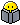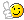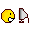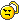# Subnetting

Member Posts: 726 ■■■■□□□□□□
Here's a good site I found today that can help you understand subnetting. I"m actually kinda understanding this stuff now.http://home.rochester.rr.com/softwretrn/netplus/ipsubnet.htm
CCNP Security - DONE!
CCNP R&S - In Progress...
CCIE Security - Future...

• have you tried...

www.howtosubnet.com
Kenny

A+, Network+, Linux+, Security+, MCSE+I, MCSE:Security, MCDBA, CCNP, CCDP, CCSP, CCVP, CCIE Written (R/S, Voice),INFOSEC, JNCIA (M and FWV), JNCIS (M and FWV), ENA, C|EH, ACA, ACS, ACE, CTP, CISSP, SSCP, MCIWD, CIWSA
• Once you get it, subnetting is really quite simple.

Here's what works for me:

Generally, just subtract whatever the last octet value is from 256 = block size. Subtract 2 (network address and broadcast address) for the number of hosts.

Example:
subnet 255.255.255.224, 256-224=32 = block size, number of hosts=30 and you can easily figure out the network and broadcast addresses from there for any subnet.

Commit these to memory:

128 192 224 240 248 252 254 255= subnet value
128 64 32 16 8 4 2 1 = binary value
1 1 1 1 1 1 1 1

AND:
2^1=2
2^2=4
2^3=8
2^4=16
2^5=32
2^6=64
2^7=128
2^8=256

So, take the number in the last octet (other than Zero) - in this case 224 - which is 3 bits turned on - so take 2^3=8 and subtract 2 and you get the number of subnets.

Why subtract 2? Because the All Zeros and All Ones subnets are ruled out (for CCNA purposes anyway.) This makes the first and last ones unusable.

There are a couple other things to know - but this is subnetting in a nutshell.
• Excellent posts! Thanks.
• Member Posts: 726 ■■■■□□□□□□
Thanks man!!!I think I'm somewhat getting it now. Numbers and I never got along too well.CCNP Security - DONE!
CCNP R&S - In Progress...
CCIE Security - Future...